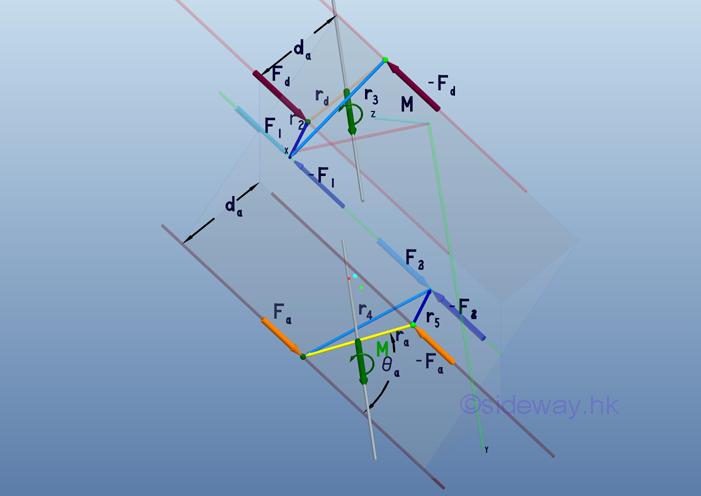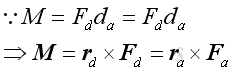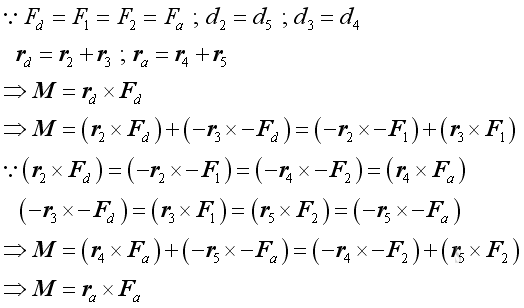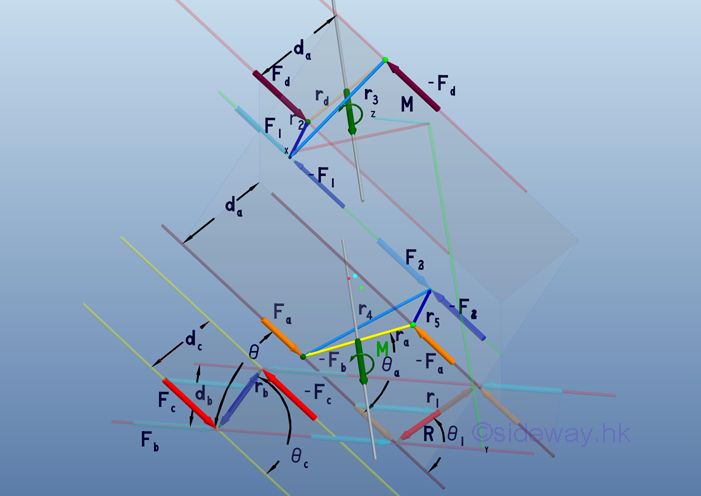output.to from Sideway
Draft for Information Only

# Content

```Equivalent Couples  Transformation of same couple in parallel planes  Couples Transformation in parallel planes```

# Equivalent Couples

Equivalent couples can also be formed by the combination of simple transformations.

## Transformation of same couple in parallel planes

Two parallel couples of same force magnitude and same prependicular distance between the lines of action in two parallel plane are equal when the force couples can be transfromed by simple couple translation, or forces and couple transformation in space.Since the two couples have the same magnitudes of forces Fa and  Fd and the prependicular distance between the forces are the same also, by definition, the effective moment of both couples of forces   Fa and  Fd can be expressed as:For parallel force couples, the lines of actions of forces are parallel also. In order to transform the couple of force Fd to the couple of force Fa  , two planes are contstructed to link up forces of two couples. One plane contains force Fd  and force -Fa  while another plane contains  force -Fd  and force Fa . Since these two planes are always crossed with each other, the line of intersection is alway parallel to the lines of action of forces.

Geometrically the intersection line will lie between the half way of the two planes because of the same prependicular distance between parallel forces, Therefore the distance between the intersection line, and the line of action of force  Fd  or of  force -Fa  are the same. And the distance between the intersection line, and the line of action of force  -Fd  or of  force Fa  are the same.

By couple transformation, force F1  and force -F1 with same magnitude as force Fd  are added along the intersection line so that the the couple of force Fd can be decomposed into two couples, i.e. couple formed by  force Fd and force -F1, and couple formed by  force F1 and force -Fd with the resultant force remains unchanged.

Similarly,  force F2 , and force -F2 with same magnitude as force F1 or force Fa  are also added along the intersection line for the couple transformation of force Fa. into two couples, i.e.  couple formed by  force Fa and force -F2, and couple formed by  force F2 and force -Fa with the resultant force remains unchanged.

Since couple formed by  force Fd and force -F1, and couple formed by  force F2 and force -Fa lie in the same plane, they can be proved that they have same moment with the same sense by planar couple transformation. And couple formed by  force F1 and force -Fd, and couple formed by  force Fa and force -F2 lie in the same plane, they can be proved that they have same moment with the same sense by planar couple transformation also.

Couple formed by force Fa and force -F2 , and couple formed by  force F2 and force -Fa can be combined to couple of force Fa  through couple transformation by the cancelling of force -F2 and  force F2 .

Therefore, couple of force Fd  can be transformated to couple of force Fa  and they are equivalent. Imply:## Couples Transformation in parallel planes

In addition to the same parallel couples transformation in parallel planes, couples transformation in parallel planes can also be done by the additional coplanar couple transformation to prove any two couples are equivalent in parallel planes.ID: 110700002 Last Updated: 7/3/2011 Revision: 0 Ref:References

1. I.C. Jong; B.G. rogers, 1991, Engineering Mechanics: Statics and Dynamics
2. F.P. Beer; E.R. Johnston,Jr.; E.R. Eisenberg, 2004, Vector Mechanics for Engineers: StaticsHome 5

Management

HBR 3

Information

Recreation

Culture

Chinese 1097

English 339

Computer

Hardware 249

Software

Application 213

Latex 52

Manim 205

KB 1

Numeric 19

Programming

Web 289

Unicode 504

HTML 66

CSS 65

SVG 46

ASP.NET 270

OS 429

Python 72

Knowledge

Mathematics

Algebra 84

Geometry 34

Calculus 67

Engineering

Mechanical

Rigid Bodies

Statics 92

Dynamics 37

Control

Natural Sciences

Electric 27